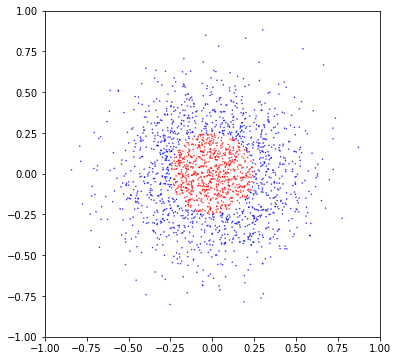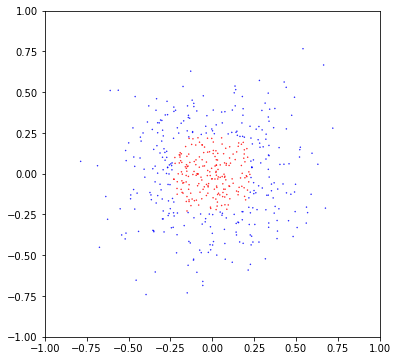# Hands-On: Simple Multi-Layer Perceptron¶

### AI and Predictive Analytics in Data-Center Environments - http://dcai.bsc.es¶

We will now see how to implement a simple MultiLayer Perceptron (MLP) usingSklearn, before applying distribution technologies.

## Generating the Data¶

For this example we will generate a simple dataset to train our first BigDL full fledged neural network. Let's start by importing the necessary packages for number manipulation and visualization and setting up a seed for the RGN:

In :
import numpy as np
import numpy.random as rd
import pandas as pd
import matplotlib.pyplot as plt
import seaborn as sns

# Set up a seed for the RNG
seed = 25740565
rd.seed(seed)


We would like to see how the neuralnetwork performs in non-linearly separable data, so we will generatetwo group of points in a radial space:

In :
# Define data parameters
num_features = 2
num_classes = 2
num_samples = 2000

mean = 0.0
var = 0.26
threshold = 0.25

x = rd.normal(mean, var, num_samples)
y = rd.normal(mean, var, num_samples)
labels = np.array([np.linalg.norm(s) > threshold for s in zip(x,y)])
features=np.vstack((x,y)).T


This data is obviously non-separable by a logisticregressionor linear models(without transforming the feature vectors xand y). We can plot it tosee that it is not linearly separable:

In :
plt.figure(figsize=(6,6))
colors = ['r', 'b']
plt.scatter(x,y, c=[colors[int(l)] for l in labels], s=0.2);
plt.xlim(-1,1); plt.ylim(-1,1);Now we create a training dataset and a test dataset for validation purposes:

In :
from sklearn.model_selection import train_test_split
features_train, features_test, labels_train, labels_test = train_test_split(features, labels, random_state=seed)


Now we are training the model. We are using the fitfunction to fit a model to the data. We are selecting a MultiLayer Perceptron, and we choose a single layer with 64 neurons:

In :
from sklearn.neural_network import MLPClassifier
mlp = MLPClassifier(hidden_layer_sizes=(64))
mlp.fit(features_train, labels_train);

/home/fjjm/.local/share/virtualenvs/exercises-oJA80Xyq/lib/python3.7/site-packages/sklearn/neural_network/_multilayer_perceptron.py:571: ConvergenceWarning: Stochastic Optimizer: Maximum iterations (200) reached and the optimization hasn't converged yet.
% self.max_iter, ConvergenceWarning)


Time to test! We can use the function predictto pass new data (here the testdataset) through the model, then we get the confusion matrix. As we have 2 classes, the confusion matrix will be a 2 x 2 table, with “Real vs Predicted”values. We want to have all the big numbers on the diagonal (“Real Red& Predicted Red”, “Real Blue& Predicted Blue”):

In :
from sklearn.metrics import classification_report,confusion_matrix
predictions = mlp.predict(features_test)
print(confusion_matrix(labels_test,predictions))

[[190  12]
[  6 292]]


As we see, the network learns pretty well, as we have around 97% of values correctly predicted. We can also print the summary of the classification, and see the accuracy and precision values:

In :
print(classification_report(labels_test,predictions))

              precision    recall  f1-score   support

False       0.97      0.94      0.95       202
True       0.96      0.98      0.97       298

accuracy                           0.96       500
macro avg       0.96      0.96      0.96       500
weighted avg       0.96      0.96      0.96       500



We can finish by plotting how the test points are classified “red”or”blue”, and see that follow the same color than the shape we used to design the dataset:

In :
plt.figure(figsize=(6,6))
plt.scatter(features_test.T, features_test.T, c=[colors[int(l)] for l in predictions], s=0.2);
plt.xlim(-1,1); plt.ylim(-1,1);